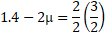# A boy is pushing a ring of mass 2 kg and radius 0.5 m with a stick as shown in the figure. The stick applies a force of 2 N on the ring and rolls it without slipping with an acceleration of. The coefficient of friction between the ground and the ring is large enough that rolling always occurs and the coefficient of friction between the stick and the ring is. The value ofis## Question ID - 101434 :- A boy is pushing a ring of mass 2 kg and radius 0.5 m with a stick as shown in the figure. The stick applies a force of 2 N on the ring and rolls it without slipping with an acceleration of. The coefficient of friction between the ground and the ring is large enough that rolling always occurs and the coefficient of friction between the stick and the ring is. The value ofis3537

(4)

Note : If net force applied by the rod is considered to be 2 N(i)From Eqs. (i) and (ii),From Eq. (ii),Note: But if only normal reaction applied by the rod is considered to be 2 N

II Law(i)(ii)Next Question :

A uniform rodof mass 2 kg is hinged at one end.The rod is kept in the horizontal position by a massless string tied to point. Find the reaction of the hinge (in N) on endof the rod at the instant when string is cut